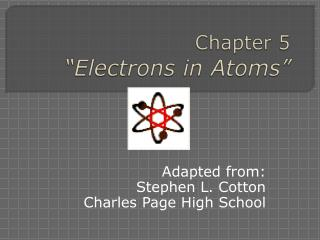DownloadDownload PresentationChapter 5 “Electrons in Atoms”

# Chapter 5 “Electrons in Atoms”

Télécharger la présentation## Chapter 5 “Electrons in Atoms”

- - - - - - - - - - - - - - - - - - - - - - - - - - - E N D - - - - - - - - - - - - - - - - - - - - - - - - - - -
##### Presentation Transcript

1. Chapter 5“Electrons in Atoms” Adapted from: Stephen L. Cotton Charles Page High School

2. Chapter 5.2Models of the Atom • OBJECTIVES: • Compare the Bohr and quantum mechanical models of the atom. • Define a quantum of energy, and explain how it is related to an energy change in matter.

3. Ernest Rutherford’s Model • Discovered dense positive piece at the center of the atom- “nucleus” • Electrons would surround and move around it, like planets around the sun • Atom is mostly empty space • It did not explain the chemical properties of the elements – a better description of the electron behavior was needed

4. Niels Bohr’s Model • Why don’t the electrons fall into the nucleus? • Move like planets around the sun. • In specific circular paths, or orbits, at different levels. • An amount of fixed energyseparates one level from another.

5. The Bohr Model of the Atom I pictured the electrons orbiting the nucleus much like planets orbiting the sun. However, electrons are found in specific circular paths around the nucleus, and can jump from one level to another. Niels Bohr

6. Bohr’s model • Energy level of an electron • analogous to the rungs of a ladder • The electron cannot exist between energy levels, just like you can’t stand between rungs on a ladder • Aquantum of energy is the amount of energy required to move an electron from one energy level to another

7. The Quantum Mechanical Model • Energy is “quantized” - It comes in chunks. • A quantum is the amount of energy needed to move from one energy level to another. • Since the energy of an atom is never “in between” there must be a quantum leap in energy. • In 1926, Erwin Schrodinger derived an equation that described the energy and position of the electrons in an atom

8. Schrodinger’s Wave Equation Equation for the probability of a single electron being found along a single axis (x-axis) Erwin Schrodinger Erwin Schrodinger

9. The Quantum Mechanical Model • Things that are very small behave differently from things big enough to see. • The quantum mechanical modelis a mathematical solution • It is not like anything you can see (like plum pudding!)

10. The Quantum Mechanical Model • Has energy levels for electrons. • Orbits are not circular. • It can only tell us the probability of finding an electron a certain distance from the nucleus.

11. Heisenberg uncertainty principle Impossible to know where an electron is located or where it is headed Scientist can not trace a path or orbit of an electron Scientist can determine the probability for an electron to occupy a certain region around the nucleus.

12. Atomic Orbitals Principal Quantum Number(n) = the energy level of the electron: 1, 2, 3, etc. Within each energy level, the complex math of Schrodinger’s equation describes several shapes. These are called atomic orbitals(coined by scientists in 1932) - regions where there is a high probability of finding an electron. Sublevels- like theater seats arranged in sections: letters s, p, d, and f

13. The modern model of atoms • Electrons are attracted to the positively charged nucleus. • Electrons are negatively charged and repel each other. • To keep electrons apart and minimize repulsions and interaction energy, • Electrons are found in principal energy levels (1, 2, 3…) • Principal energy levels are divided into sublevels

14. The modern model of atoms Electron cloud a.Thesublevels are called s, p, d,and fin order of increasing energy b. Each sublevel is divided into orbitalsthat can contain 2 electrons. c. Orbitals within a sublevel have equal energy d. Orbitals are regions of space. Electrons stay in their orbital 90% of the time.

15. The modern model of atoms Principal energy levels Placement of electrons in an atom Sublevel # orbitals # electrons s 1 2 p 3 6 d 5 10 f 7 14 2 1 repel Electron cloud

16. contains contains contains The modern model of atoms Summary Electron cloud Principal energy levels (1, 2, 3…) Energy sublevels (s, p, d, f) Orbitals(up to 2 electrons) Principal energy levels 2 1 repel Electron cloud

17. First Energy Level Has only s orbital only 2 electrons 1s2 Second Energy Level Has s and p orbitals available 2 in s, 6 in p 2s22p6 8 total electrons By Energy Level

18. Third energy level Has s, p, and d orbitals 2 in s, 6 in p, and 10 in d 3s23p63d10 18 total electrons Fourth energy level Has s, p, d, and f orbitals 2 in s, 6 in p, 10 in d, and 14 in f 4s24p64d104f14 32 total electrons By Energy Level

19. Any more than the fourth and not all the orbitals will fill up. You simply run out of electrons The orbitals do not fill up in a neat order. The energy levels overlap Lowest energy fill first. By Energy Level